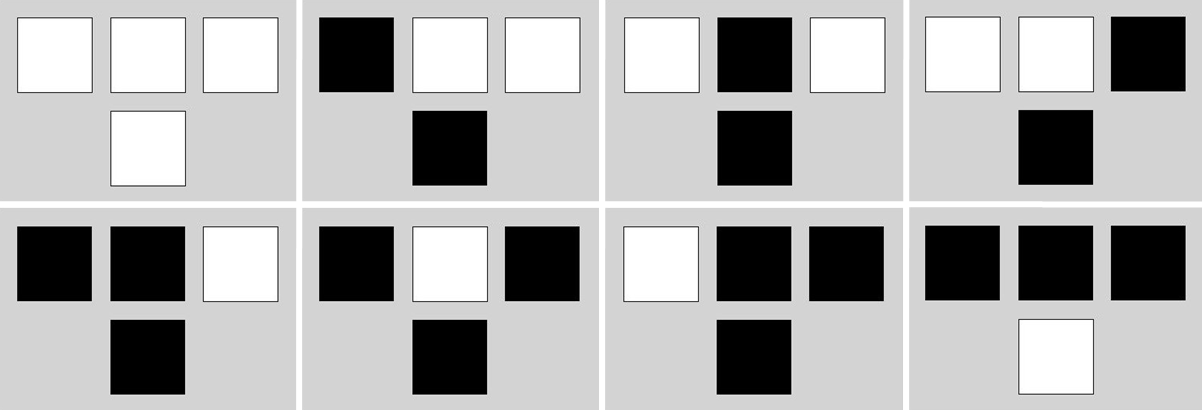# Cellular Automata: The Beauty Of Simplicity

I am strangely attracted to you (Cole Porter)

Imagine a linear grid that extends to the left and right. The grid consists of cells that may be only one of these two states: On or Off. At each time step, the next state of a cell is computed as a function of its left and right neighbors and the current state of the cell itself. Given one cell, if the three cells (both inmediate neighbors and cell itself) are on or off, next state of the cell is Off. Otherwise, next state is On. These simple rules can be represented graphically as follows, where white colour is equal to Off and black one is equal to On:Lets assume that time flows in a downward direction; thus, the cell inmediately below another cell represents the next sate. Last assumption: cells on one edge are neighbors of the cells of the opposite edge. Whe have defined a One-Dimensional Cellular Automata with finite states.

This is what happens when we initialize as Off all cells except for the two center cells, initialized as On:

Previous plots represent time evolution of the automata for 8, 16, 32 and 64 degress of time (i.e. depth). And this is the result for 256 degrees:Result is very similar to the well known Sierpinski triangle. And this is what happens when you initialize cells ramdomly:I like a lot the small triangles that appears as automata evolves. I am strangely attracted to them.

Here you have the code to build triangle:

```library(sp)
width <-2^5
depth <-width/2
gt = GridTopology(cellcentre=c(1,1),cellsize=c(1,1),cells=c(width, depth))
gt = SpatialGrid(gt)
z <- data.frame(status=sample(0:0, width, replace=T))
z[width/2, 1] <- 1
z[width/2+1, 1] <- 1
for (i in (width+1):(width*depth))
{
ilf <- i-width-1
iup <- i-width
irg <- i-width+1
if (i%%width==0) irg <- i-2*width+1
if (i%%width==1) ilf <- i-1
if((z[ilf,1]+z[iup,1]+z[irg,1]>0)&(z[ilf,1]+z[iup,1]+z[irg,1]<3))
{st <- 1} else {st <- 0}
nr<-as.data.frame(st)
colnames(nr)<-c("status")
z<-rbind(z,nr)
}
sgdf = SpatialGridDataFrame(gt, z)
image(sgdf, col=c("white", "black"))
```